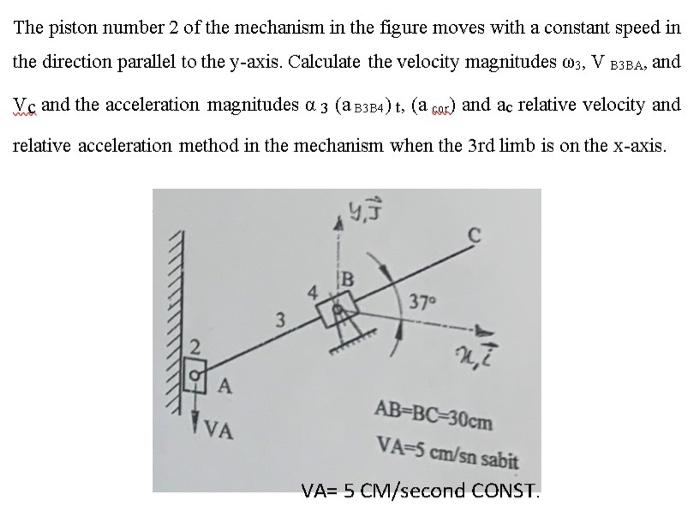# (Solved): mechanismplease provide the answer as a photo with the steps The piston number 2 of the mechanism in ...

mechanismThe piston number 2 of the mechanism in the figure moves with a constant speed in the direction parallel to the y-axis. Calculate the velocity magnitudes $$\omega_{3}, V_{\text {??? }}$$, and $$\mathrm{V}_{c}$$ and the acceleration magnitudes $$\alpha_{3}\left(\mathrm{a}_{\mathrm{B} 3 \mathrm{~B}}\right) \mathrm{t},\left(\mathrm{a}_{\mathrm{Gar}}\right)$$ and $$\mathrm{ac}_{\mathrm{c}}$$ relative velocity and relative acceleration method in the mechanism when the 3rd limb is on the $$\mathrm{x}$$-axis.

We have an Answer from Expert